2019-03-20 17:09:24 qq_32903613 阅读数 953
• ###### Python数据分析实战-Pandas

深度学习、机器学习和数据分析必须用pandas。pandas是在python直接流行的数据处理框架。可以说，如果不会使用pandas，就谈不上会用python做数据分析。本课程会使用奥林匹克一个真实的数据作为实验数据，从初级到各种pandas的常用操作，到常用的数据可视化，让你在短的时间内掌握好pandas，轻松愉快的玩转数据分析。

2342 人正在学习 去看看 阿勒拉哈

``````from math import ceil

import pandas as pd
import pymysql

def getDataFromMysql():
"""
连接数据库

"""

conn = pymysql.connect(host='127.0.0.1', user="xxx", passwd="xxx", db="xxx", port=3306, charset="utf8")
cur = conn.cursor()
cur.execute("select * from movies")
movies = cur.fetchall()
cur.close()
conn.close()

return movies

def dataFrame2sheet(dataframe, excelWriter, countn):
"""
pandas dataframe生成excel

"""
# DataFrame转换成excel中的sheet表
dataframe.to_excel(excel_writer=excelWriter, sheet_name="info_{0}".format(countn), index=None)

excelWriter.save()
excelWriter.close()

"""
excel中新增sheet表
"""

"""
在excel中新增sheet表
"""
excelWriter.book = book

dataframe.to_excel(excel_writer=excelWriter, sheet_name="info_{0}".format(i), index=None)

excelWriter.close()

if __name__ == '__main__':

movies = getDataFromMysql()
dataSet = []
for i in movies:
dataSet.append(list(i))

# excelPath
excelPath = "./test.xlsx"

# 生成DataFrame
countn = ceil(len(dataSet) / 200)
if countn <= 1:

dataframe = pd.DataFrame(dataSet[:200])

# 创建ExcelWriter 对象
excelWriter = pd.ExcelWriter(excelPath, engine='openpyxl')

# 生成excel

dataFrame2sheet(dataframe, excelWriter, countn)

# excel中增加sheet
else:
# 使用公共的excelWriter，数据不会被覆盖
excelWriter = pd.ExcelWriter(excelPath, engine='openpyxl')
for i in range(1, countn + 1):
dataframe = pd.DataFrame(dataSet[(i - 1) * 200:i * 200])

# 在excel里增加sheet，并写入数据。这个也可以实现

# 生成excel
dataFrame2sheet(dataframe, excelWriter, countn=i)
``````

pandas 导出大数据 相关内容

2020-01-07 20:55:16 jp_666 阅读数 8
• ###### Python数据分析实战-Pandas

深度学习、机器学习和数据分析必须用pandas。pandas是在python直接流行的数据处理框架。可以说，如果不会使用pandas，就谈不上会用python做数据分析。本课程会使用奥林匹克一个真实的数据作为实验数据，从初级到各种pandas的常用操作，到常用的数据可视化，让你在短的时间内掌握好pandas，轻松愉快的玩转数据分析。

2342 人正在学习 去看看 阿勒拉哈

pandas导出数据到文件的四种方式

``````import pandas as pd
import pymysql

df = pd.DataFrame({'A': [3, 4, 8, 9], 'B': [1.2, 2.4, 4.5, 7.3], 'C': ["aa", "bb", "cc", "dd"]})

def export_data_to_csv():
# 参数encoding="utf_8_sig"编码后，可以防止写入csv的中文出现乱码
df.to_csv("./test.csv", encoding="utf_8_sig")

def export_data_to_excel():

def export_data_to_table():
use_unicode=True)
df.to_sql(name='table_name', con=con, if_exists='append', index=False)

def export_data_to_json():
df.to_json("test.txt")

def main():
export_data_to_csv() # 导出数据到CSV文件
export_data_to_excel() # 导出数据到Excel文件
export_data_to_table() # 导出数据到SQL表
export_data_to_json() # 以Json格式导出数据到文本文件

if __name__ == '__main__':
main()
``````pandas 导出大数据 相关内容

2019-01-08 16:08:36 qq_36636519 阅读数 810
• ###### Python数据分析实战-Pandas

深度学习、机器学习和数据分析必须用pandas。pandas是在python直接流行的数据处理框架。可以说，如果不会使用pandas，就谈不上会用python做数据分析。本课程会使用奥林匹克一个真实的数据作为实验数据，从初级到各种pandas的常用操作，到常用的数据可视化，让你在短的时间内掌握好pandas，轻松愉快的玩转数据分析。

2342 人正在学习 去看看 阿勒拉哈

### 一、Pandas导入数据

导入数据类型 说明
pd.DataFrame(dict) # 从字典对象导入数据，Key是列名，Value是数据

``````pd.read_csv(filepath_or_buffer, sep=', ', delimiter=None, header='infer', names=None,
index_col=None, usecols=None,dtype=None,
skipinitialspace=False,
skiprows=None, nrows=None, na_filter=True,iterator=False, chunksize=None,  encoding=None, low_memory=True)
``````
• filepath_or_buffer : str，pathlib。str, pathlib.Path, py._path.local.LocalPath or any object with a read() method (such as a file handle or StringIO)
可以是CSV文件，也可以是URL，可用URL类型包括：http, ftp, s3等。
• 分割符设置参数 ： sep,delimiter,delim_whiterspace

**sep ?*str,default ‘,’
指定分隔符。如果不指定参数，默认分隔符为‘,’
**delimiter : ** str,default None
定界符，备选分隔符，作用与seq一样，它的优先级比seq高，因此如果指定该参数，则sep参数失效
**delim_whitespace : ** boolean, default False.
指定空格(例如’ ‘或者’ ‘)是否作为分隔符使用，等效于设定sep=’\s+’。如果这个参数设定为Ture那么delimiter 参数失效。在新版本0.18.1支持

**header ：**int or list of ints, default ‘infer’
names ： array-like, default None
index_col ： int or sequence or False, default None
index_col作用是设置行索引的值
默认index_col= None ,即行索引由系统自动生成,[0,1,2,3,4,5…]
index_col= 0 : 表示由第一列值作为行索引，同理 1 是第二列值作为行索引
也可以用列名设置 : index_col = “name” 表示由 name这列作为行索引

• encoding ： 设置文件编码格式，默认是utf-8,如果导入的文件编码格式非utf-8,需要手动设置，否则会报错。
• dtype : Type name or dict of column -> type, default None
每列数据的数据类型。例如 {‘a’: np.float64, ‘b’: np.int32}
• skiprows: list-like or interger,default None
需要忽略的行数（从文件开始处算起），或需要跳过的行号列表（从0开始）
skiprows = 1 ：表示忽略第一行，从第二行开始导入数据，该参数可以用来替代skip_blank_lines，将空行忽略的作用。
skiprows = [0,2,4,8] ：跳过0,2,4,8行，其他数据导入Pandas中
• usecols: 读取csv文件的指定列
• skiprows: list-like or integer, default None
需要忽略的行数（从文件开始处算起），或需要跳过的行号列表（从0开始）。
• na_filter: boolean, default True
是否检查丢失值（空字符串或者是空值）。对于大文件来说数据集中没有空值，设定na_filter=False可以提升读取速度。
• skip_blank_lines：boolean, default True
如果为True，则跳过空行；否则记为NaN。
• usecols：array-like, default None
返回一个数据子集，该列表中的值必须可以对应到文件中的位置（数字可以对应到指定的列）或者是字符传为文件中的列名。例如：usecols有效参数可能是 [0,1,2]或者是 [‘foo’, ‘bar’, ‘baz’]。使用这个参数可以加快加载速度并降低内存消耗。
• chunksize：int, default None
• low_memory：boolean, default True
分块加载到内存，再低内存消耗中解析。但是可能出现类型混淆。确保类型不被混淆需要设置为False。或者使用dtype 参数指定类型。注意使用chunksize 或者iterator 参数分块读入会将整个文件读入到一个Dataframe，而忽略类型（只能在C解析器中有效）

2、pd.DataFrame()

``````pd.DataFrame(data=None, index=None, columns=None, dtype=None, copy=False)
``````
• data: numpy ndarray (structured or homogeneous), dict, or DataFrame
Dict can contain Series, arrays, constants, or list-like objects
Changed in version 0.23.0: If data is a dict, argument order is maintained for Python 3.6 and later.

data参数可以传入多维数组、字典、或者DataFrame类型；字典类型可以包含Series、arrays

创建DataFrame的方法：
1、通过单个Series 对象创建
2、通过字典列表创建
3、通过Series对象字典创建
4、通过Numpy二维数组创建

``````创建 DataFrame

import pandas as pd
area_dict = {"Califotnia":423967,"Texas":695662,"New Tork":141297,'Florida':170312,'Illinois':149995}
area = pd.Series(area_dict)

popu_dict = {"Califotnia":38332521,"Texas":26448193,"New Tork":19651127,'Florida':19552860,'Illinois':12882135}
population = pd.Series(popu_dict)

# 方法一 ：通过单个DataFrame对象创建
pd.DataFrame(population,columns=["populations"])
populations
Califotnia     38332521
Florida        19552860
Illinois       12882135
New Tork       19651127
Texas          26448193

# 方法二： 通过 多个Series对象字典创建
states = pd.DataFrame({'population':population,"area":area})

states
area  population
Califotnia  423967    38332521
Florida     170312    19552860
Illinois    149995    12882135
New Tork    141297    19651127
Texas       695662    26448193

# 方法三 : 通过字典列表创建 任何元素是字典的列表都可以变成 DataFrame
data = [{"a":i,"b":2*i} for i in range(3) ]

data
[{'a': 0, 'b': 0}, {'a': 1, 'b': 2}, {'a': 2, 'b': 4}]

pd.DataFrame(data)
a  b
0  0  0
1  1  2
2  2  4

方法四 :  通过Numpy 二维数组创建，假如有一个二维数组，就可以创建一个可以指定行列索引值的 DataFrame 。如果不指定行列索引值，那么行列默认都是整数索引值。
arr = np.random.randint(10,size=(2,3))
arr
array([[6, 9, 9],
[6, 3, 7]])

pd.DataFrame(arr,index=[1,2],columns=["A","B","C"])
A  B  C
1  6  9  9
2  6  3  7

``````

### 二、Pandas导出数据

导出数据类型 说明
df.to_csv(filename) 导出数据到CSV文件
df.to_excel(filename) 导出数据到Excel文件
df.to_sql(table_name, connection_object) 导出数据到SQL表
df.to_json(filename) 以Json格式导出数据到文本文件
• to_csv()
``````to_csv(path_or_buf=None, sep=',', na_rep='', float_format=None, columns=None, header=True, index=True, index_label=None, mode='w', encoding=None, compression=None, quoting=None, quotechar='"', line_terminator='\n', chunksize=None, tupleize_cols=None, date_format=None, doublequote=True, escapechar=None, decimal='.')
``````
• sep：分隔符 Field delimiter for the output file (default ”,”)
默认使用","作为分割符
• na_rep：替换空值 A string representation of a missing value (default ‘ ’)
缺失值默认保存为空，可以用其他字符替换空字符
• columns：列 sequence,optional Columns to write
设置columns=[“name”,“hight”] 表示设置提取DataFrame中的name和hight列保持到文件中

header： boolean or list of string, default True
是否输出列名。如果给定字符串列表，则假定为列名的别名的结果来输出
index： boolean, default True
表示是否显示行索引，默认为True ；index=None表示设置行索引不显示

• float_format : 设置浮点数输出格式
dt.to_csv(‘C:/Users/think/Desktop/Result1.csv’,float_format=’%.2f’) #保留两位小数
``````data3.to_csv("data3test2.csv",sep=',',na_rep='null',columns=["name","hight"],header=None,index=None)
``````

### 三、Pandas检查数据

Pandas 检查数据的方法 说明
df.tail(n) 查看DataFrame对象的最后n行
df.shape() 查看行数和列数
df.info() 查看索引、数据类型和内存信息
df.describe() 查看数值型列的汇总统计
s.value_counts(dropna=False) 查看Series对象的唯一值和计数
df.apply(pd.Series.value_counts) 查看DataFrame对象中每一列的唯一值和计数

### 四、Pandas统计数据

Pandas 统计数据的方法 说明
df.describe() # 查看数据值列的汇总统计
df.mean() 返回所有列的均值
df.corr() 返回列与列之间的相关系数
df.count() 返回每一列中的非空值的个数
df.max() 返回每一列的最大值
df.min() 返回每一列的最小值
df.median() 返回每一列的中位数
df.std() 返回每一列的标准差

pandas 导出大数据 相关内容

2019-03-31 16:35:43 muyao987 阅读数 1590
• ###### Python数据分析实战-Pandas

深度学习、机器学习和数据分析必须用pandas。pandas是在python直接流行的数据处理框架。可以说，如果不会使用pandas，就谈不上会用python做数据分析。本课程会使用奥林匹克一个真实的数据作为实验数据，从初级到各种pandas的常用操作，到常用的数据可视化，让你在短的时间内掌握好pandas，轻松愉快的玩转数据分析。

2342 人正在学习 去看看 阿勒拉哈

参考教程：点这里

目录

pandas的series和dataFrame

pandas选择数据

pandas设置值

pandas处理丢失数据NaN

pandas导入导出

pandas数据合并

pandas结合plot绘图

END

### pandas的series和dataFrame

pandas和numpy的关系：numpy是列表，pandas是字典，pandas基于numpy构建。

Series的形式：索引在左边，值在右边。没有为数据指定索引会自动创建0到N-1（N为长度）的整数型索引。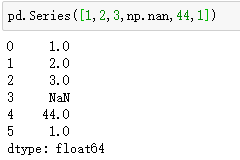DataFrame是一个表格型的数据结构，每列可以是不同的值类型，既有行索引也有列索引。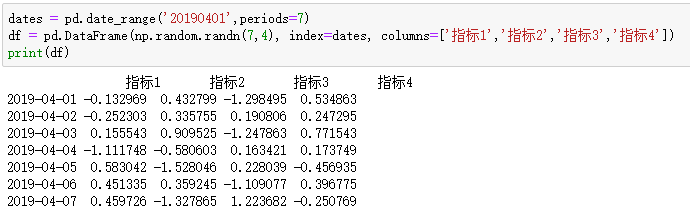取df的“指标1”列：df['指标1']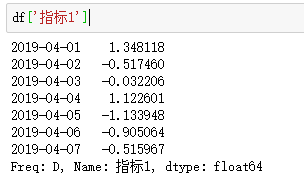创建一组没有给定行标签和列标签的数据：pd.DataFrame(np.arange(12).reshape((3,4)))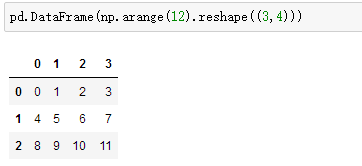创建对每一列的数据进行特殊对待的数据：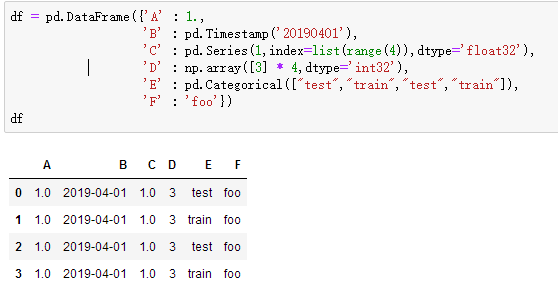查看数据类型：df.dtypes

查看索引（行）的序号：df.index

查看每种数据（列）的名：df.columns

查看df的所有值：df.values

查看数据的总结（计数、平均值、最值等）：df.describe()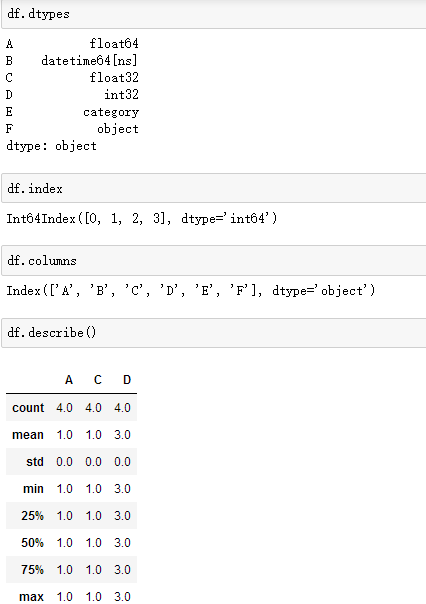翻转数据：df.T

对数据的 index 进行排序：df.sort_index(axis=1, ascending=False)

对数据的 进行排序：df.sort_values(by='A')

### pandas选择数据

选择某列A： df['A'] 或 df.A

选择0到2多列：df[0:2]

根据标签选择1行：df.loc 【选择索引为0的一行】

选择所有行（: 代表所有行）：df.loc[:,['A','B']]  【选择所有行的A、B两列】

df.loc[3,['A','B']] 【选择第3行的A、B两列】

根据位置（索引）进行选择数据：df.iloc[3,1]  【第3行第1列的数据】

df.iloc[2:3,0:3]   【第2到3行，第0到3列的数据】

df.iloc[[0,3],0:3] 【第0、3行，第0到3列的数据】

通过判断的筛选：df[df.A==2]  【选择列A的值为2的行】

### pandas设置值

利用索引：df.iloc[2,2] = 1111  【修改第2行、 第2列】

利用标签：df.loc['20190401','B'] = 2222  【修改行‘20190401’、 列‘B’】

根据条件：df.B[df.A>4] = 0  【列A大于4的都改成0】

按行或列：df['F'] = np.nan  【加上新列F，并设值为NaN】

`               df_original['title_keywords'] = ['' for _ in range(content_num)]`

添加数据：df['G'] = pd.Series([1,2,3,4,5,6], index=pd.date_range('20190401',periods=6))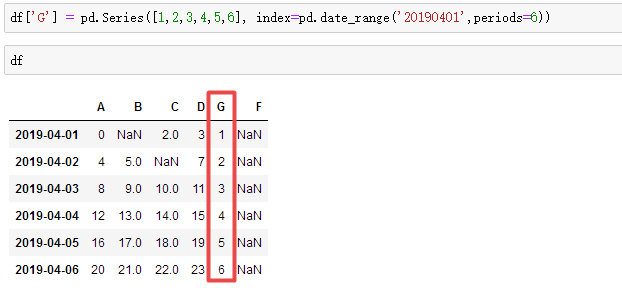### pandas处理丢失数据NaN

直接去掉有 NaN 的行或列（pd.dropna()）：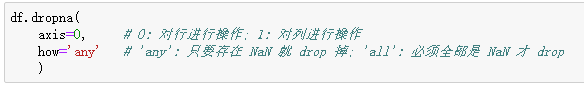将 NaN 的值用其他值代替（pd.fillna()）：df.fillna(value=0)  【全替换成0】

判断每个值是否是缺失数据：df.isnull()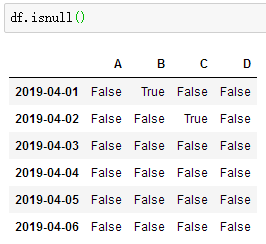检测整个数据表中是否存在 NaN, 如果存在就返回 True：np.any(df.isnull()) == True

### pandas导入导出

pandas可以读取与存取的格式：csv、excel、json、html、pickle等 【官方文档

将资料存取成pickle：data.to_pickle('student.pickle')

### pandas数据合并

concat纵向合并：res = pd.concat([df1, df2, df3], axis=0)  【可以发现索引没变】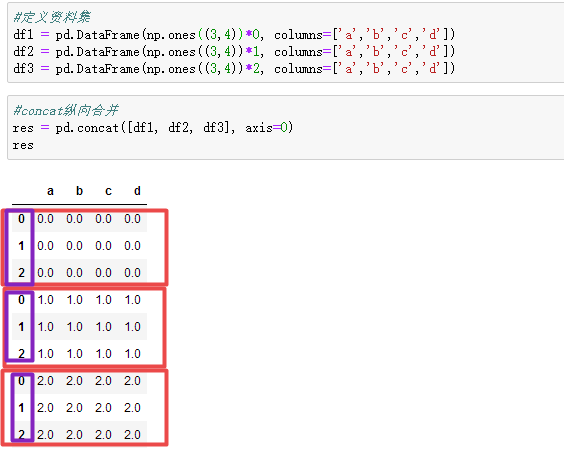concat参数之ignore_index (重置 index) ：res = pd.concat([df1, df2, df3], axis=0, ignore_index=True)

concat参数之join (合并方式，默认join='outer') ：res = pd.concat([df1, df2], axis=0, join='outer')  【依照column来做纵向合                                                                                                      并，有相同的column上下合并在一起，其他独自的column个自成列，原本                                                                                                  没有值的位置皆以NaN填充。】

concat参数之join (合并方式，改为join='inner') ：res = pd.concat([df1, df2], axis=0, join='inner')   【只有相同的column合并                                                                                                    在一起，其他的会被抛弃】

concat参数之join_axes：【根据df1的行索引来join，下图2为去掉join_axes】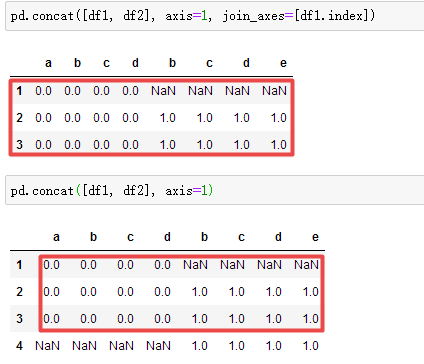append只有纵向合并，没有横向合并：

df1.append(df2, ignore_index=True)  【将df2合并到df1的下面，重置index】

df1.append([df2, df3], ignore_index=True)  【合并多个df，将df2与df3合并至df1的下面，重置index】

df1.append(s1, ignore_index=True)  【合并series，将s1合并至df1，重置index】

merge用于两组有key column的数据【样例数据如下】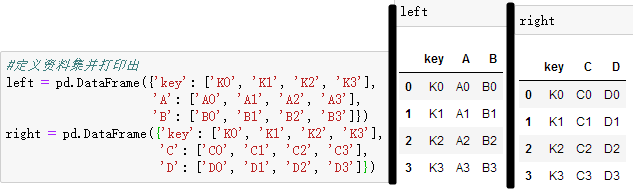依据key1与key2 columns进行合并，并打印出四种结果['left', 'right', 'outer', 'inner']：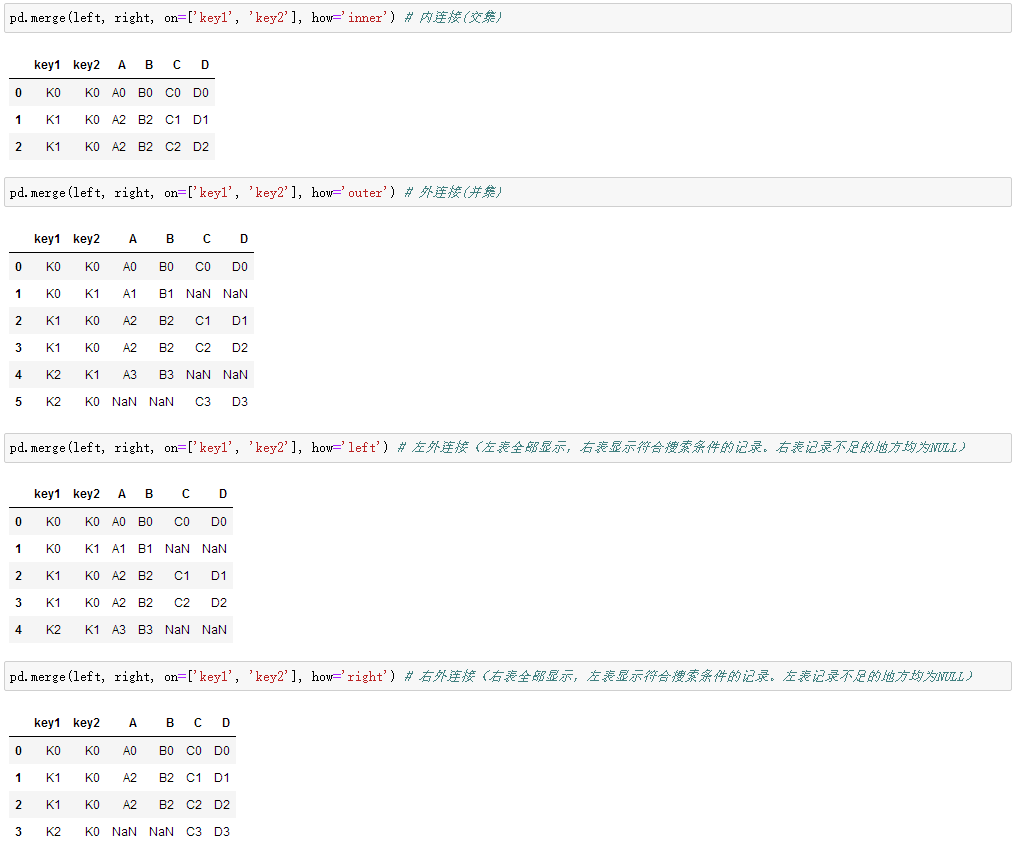merge参数之indicator=True会将合并的记录放在新的一列

merge参数之indicator=str，自定义列名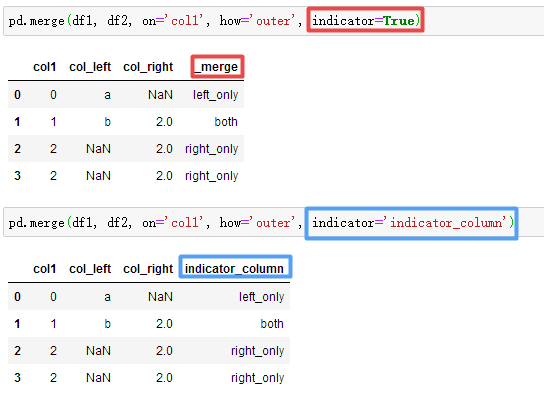根据index合并：res = pd.merge(left, right, left_index=True, right_index=True, how='outer')

merge参数之suffixes解决overlapping（重叠）的问题 【名为K0的有一个1岁男、1个4岁女、1个5岁女】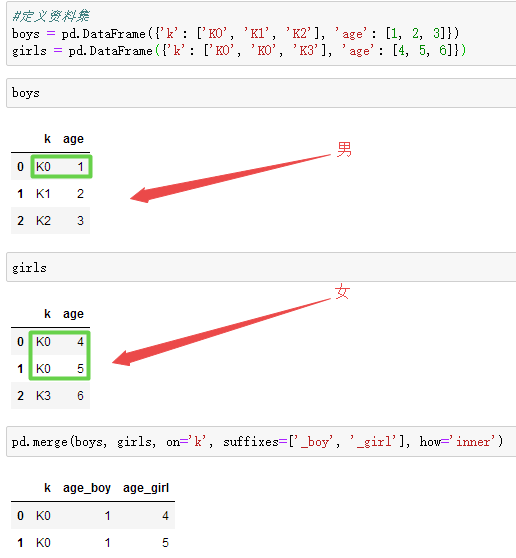### pandas结合plot绘图

``````import matplotlib.pyplot as plt
``````

随机生成1000个数据，Series 默认的 index 就是从0开始的整数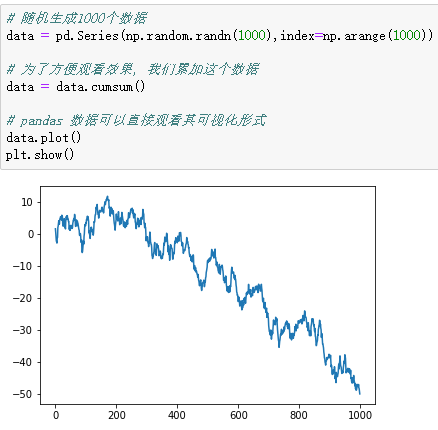生成一个1000*4 的DataFrame，并对他们累加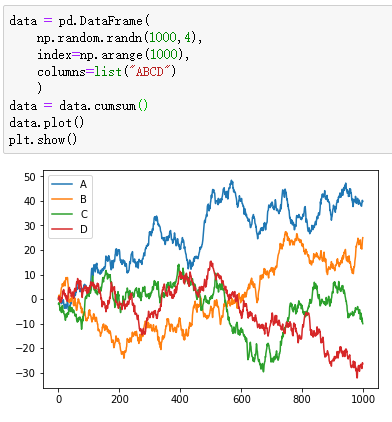散点图scatter只有x，y两个属性，我们我们就可以分别给x, y指定数据【下图line1】

再画一个在同一个ax上面，选择不一样的数据列，不同的 color 和 label【下图line2】

显示图片【下图line3】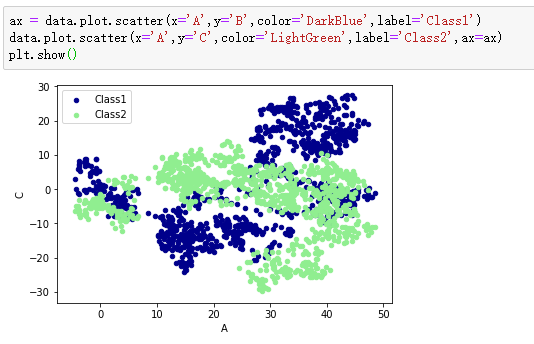### END

pandas 导出大数据 相关内容

2016-10-02 15:04:37 u012135425 阅读数 422
• ###### Python数据分析实战-Pandas

深度学习、机器学习和数据分析必须用pandas。pandas是在python直接流行的数据处理框架。可以说，如果不会使用pandas，就谈不上会用python做数据分析。本课程会使用奥林匹克一个真实的数据作为实验数据，从初级到各种pandas的常用操作，到常用的数据可视化，让你在短的时间内掌握好pandas，轻松愉快的玩转数据分析。

2342 人正在学习 去看看 阿勒拉哈

一、通过pandas导出数据

pandas的两大数据类型Series和DataFrame都有自己标准的IO方法，API中可以一一了解学习

Series和DataFrame中强大的导出功能，可以导出成各种类型的数据例子：首先创建一个DataFrame类型的数据，数据有两列，姓名和年龄

```>>> import pandas
>>> df = pandas.DataFrame({
... 'name':['Tom','张三','LinDa'],
... 'age':[23,24,22]
... })
>>> df
age   name
0   23    Tom
1   24     张三
2   22  LinDa```
接下来把这些数据存储到csv文件当中

` df.to_csv('student1.csv')#每一行都有索引`
`df.to_csv('student1.csv',index=False)#去掉索引`

pandas 导出大数据 相关内容

阅读数 1598

博文 来自： fangafangxiaoniu

阅读数 134

博文 来自： zjw18737392679

阅读数 12

博文 来自： weixin_42167712

阅读数 279

博文 来自： devcloud

阅读数 21

博文 来自： halokwas
没有更多推荐了，返回首页